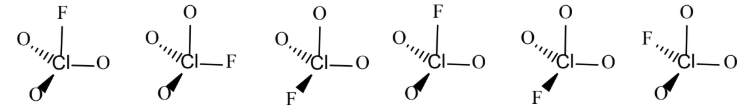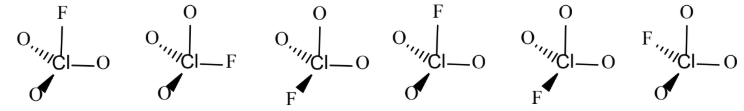# Problem: FClO3 is a tetrahedral molecule with chlorine (Cl) at the center. When FClO 3 crystallizes in the solid state, there is no preference for one orientation over another. In other words, the unique F atom ends up randomly distributed among the tetrahedral sites, as shown below.Calculate the expected residual molar entropy S for FClO 3 at 0 K. Show all work and circle your final answer.A. 2.47x10-23 J/mol•KB. 14.89 J/mol•KC. 1.91x10-23 J/mol•KD. 11.52 J/mol•KE. 0 J/mol•K

###### FREE Expert Solution

We’re being asked to calculate the expected residual molar entropy (S) for FClO3 at 0.

The possible ways for FClO3 to arrange itself and the unique F atom ends up randomly distributed among the tetrahedral sites, as shown below:92% (40 ratings)###### Problem Details

FClO3 is a tetrahedral molecule with chlorine (Cl) at the center. When FClO 3 crystallizes in the solid state, there is no preference for one orientation over another. In other words, the unique F atom ends up randomly distributed among the tetrahedral sites, as shown below.Calculate the expected residual molar entropy S for FClO 3 at 0 K. Show all work and circle your final answer.

A. 2.47x10-23 J/mol•K

B. 14.89 J/mol•K

C. 1.91x10-23 J/mol•K

D. 11.52 J/mol•K

E. 0 J/mol•K